# Percents Less Than 1 or Greater Than 100Problem 1: Glosser Grade School has an enrollment of 400 students. Only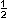% of students ride their bicycles to school each day. How many students is this?

We know from previous lessons how to write a percent as a decimal by moving the decimal point two places to the left. So we get the following:

If= 0.5, then% = 0.5%, and 0.5% =.005. Thus we get% = .005

Going back to our original problem, we get: .005 of 400 = .005 x 400 = 2

Solution: 2 students ride their bicycle to school each day.

Problem 2: This year, enrollment at Glosser Grade school is 150% of last year. How many students are enrolled now?

Writing 150% as a decimal, we get: 150% = 1.50 = 1.5

1.5 of 400 = 1.5 x 400 = 600

Solution: 600 students are now enrolled at Glosser Grade School.

In Problem 1, we were asked to work with a percent less than 1, and in Problem 2, we were asked to work with a percent greater than 100. When working with percents less than 1 and greater than 100, we follow the same rules that we learned for percents between 1 to 100. Let's look at some examples.

Example 1: Write each percent as a decimal: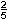%,    1.8%,    0.32%,    235%

Since= 0.4, we get% = 0.4%

 Solution Percent Decimal 0.4% 0.004 1.8% 0.018 0.32% 0.0032 235% 2.35

Note that instead of writing .32% we write 0.32%   The leading zero reminds us that this number is between 0 and 1 percent. Let's look at some more examples.

Example 2: Write each percent as a fraction in lowest terms:    0.42%,    395%,    0.07%

 Solution Percent Decimal Fraction Lowest Terms 0.42% 0.0042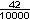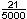395% 3.95 33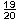0.07% 0.0007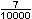Example 3: Write each decimal as a percent:    12.2,    0.00459,    1.2765

 Solution Decimal Percent 12.2 1,220% 0.00459 0.459% 1.2765 127.65%

Summary: When working with percents less than 1 and greater than 100 (special percents), we follow the same rules that we learned for percents between 1 and 100. In this lesson, we applied these rules to help us make conversions between fractions, decimals and special percents.

### Exercises

Directions: Read each question below. Select your answer by clicking on its button. Immediate feedback is provided in the RESULTS BOX. If you make a mistake, choose a different button.

 1.  Which of the following is equal to 190%? 190.0       19.0       1.90       None of the above.       RESULTS BOX:
 2.  Which of the following is equal to 0.37%? 0.0037       0.037       0.37       None of the above.       RESULTS BOX:
 3.  Which of the following is equal to 0.00319? 3.19%       0.319%       0.0319%       None of the above.       RESULTS BOX:
 4.  Which of the following is equal to 29.348? 2,934.8%       293.48%       29.348%       None of the above.       RESULTS BOX:
 5.  Which number has the least value? 0.0056       0.56%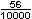All have the same value.       RESULTS BOX: